# ML | Understanding Hypothesis

In most supervised machine learning algorithm, our main goal is to find out a possible hypothesis from the hypothesis space that could possibly map out the inputs to the proper outputs.
The following figure shows the common method to find out the possible hypothesis from the Hypothesis space: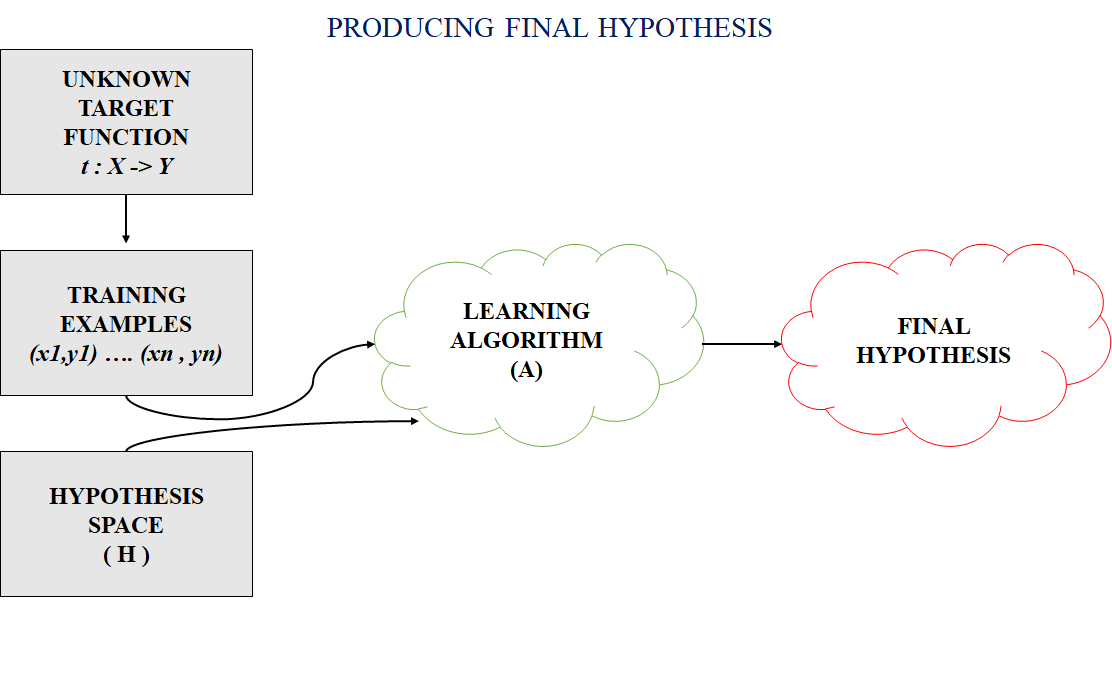Hypothesis Space (H):
Hypothesis space is the set of all the possible legal hypothesis. This is the set from which the machine learning algorithm would determine the best possible (only one) which would best describe the target function or the outputs.

Hypothesis (h):
A hypothesis is a function that best describes the target in supervised machine learning. The hypothesis that an algorithm would come up depends upon the data and also depends upon the restrictions and bias that we have imposed on the data. To better understand the Hypothesis Space and Hypothesis consider the following coordinate that shows the distribution of some data: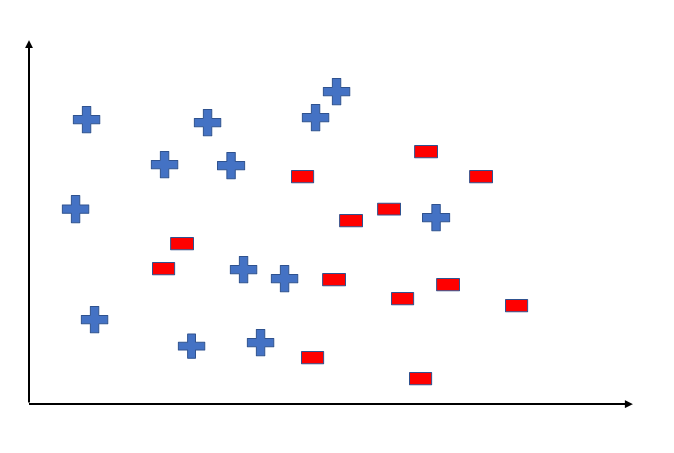Say suppose we have test data for which we have to determine the outputs or results. The test data is as shown below: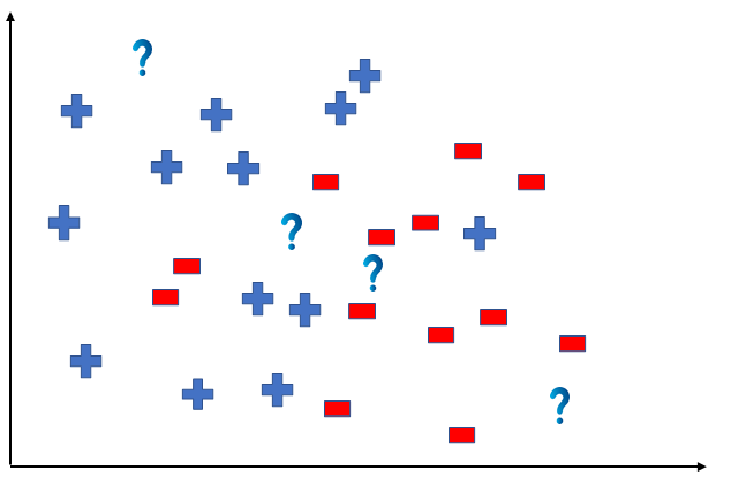We can predict the outcomes by dividing the coordinate as shown below: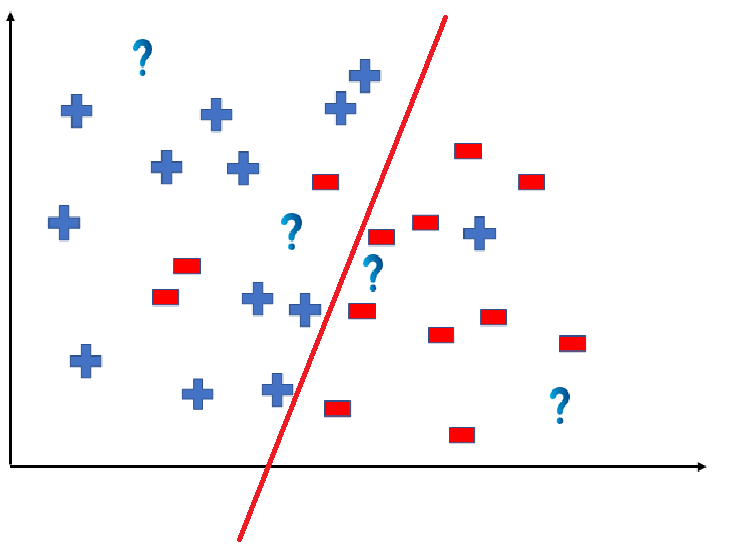So the test data would yield the following result: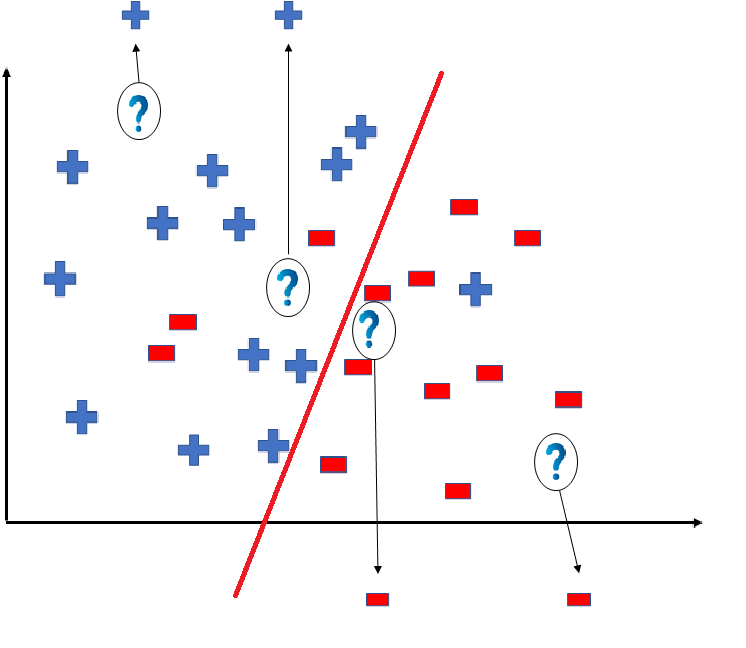But note here that we could have divided the coordinate plane as: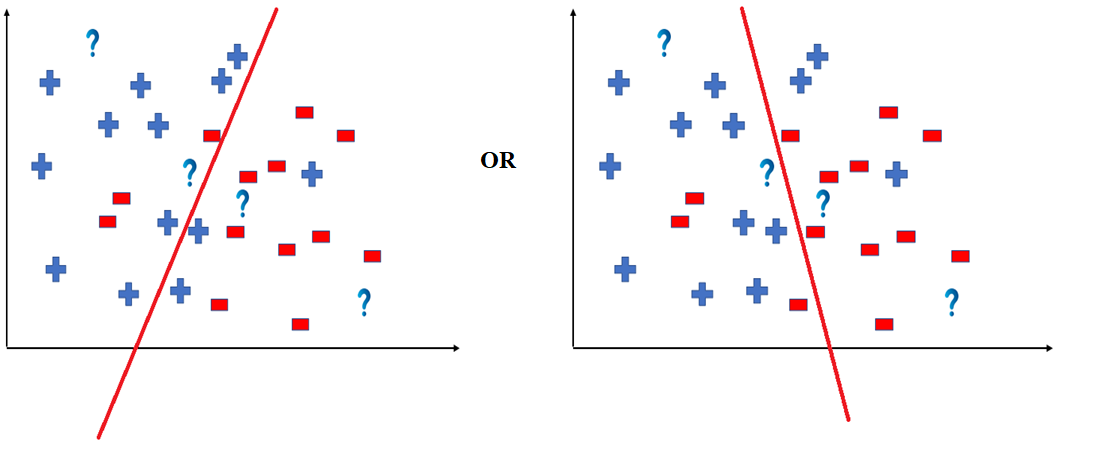The way in which the coordinate would be divided depends on the data, algorithm and constraints.

• All these legal possible ways in which we can divide the coordinate plane to predict the outcome of the test data composes of the Hypothesis Space.
• Each individual possible way is known as the hypothesis.
• Hence, in this example the hypothesis space would be like: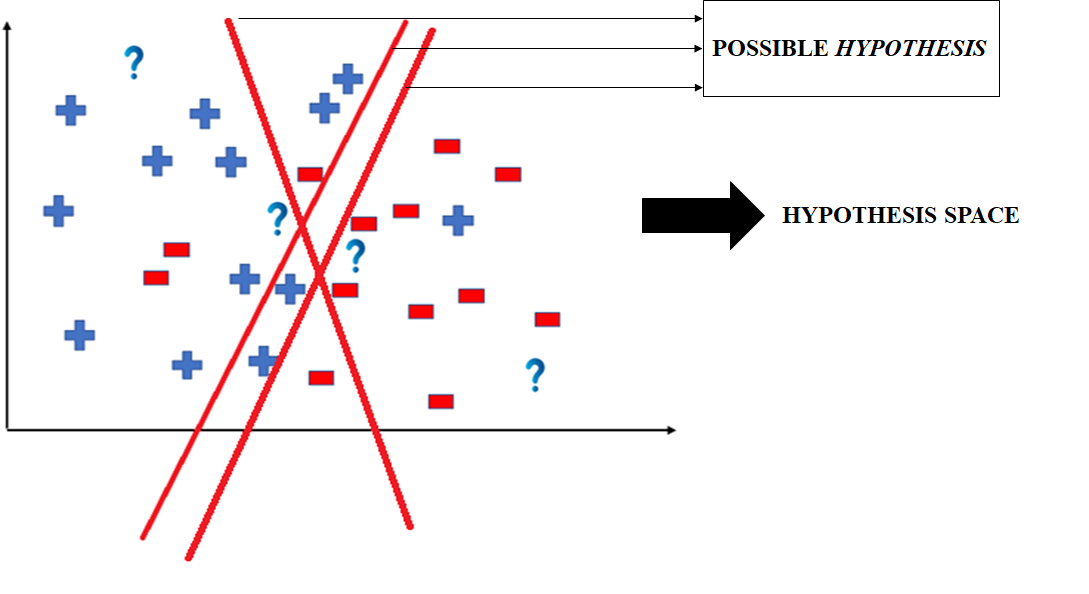Whether you're preparing for your first job interview or aiming to upskill in this ever-evolving tech landscape, GeeksforGeeks Courses are your key to success. We provide top-quality content at affordable prices, all geared towards accelerating your growth in a time-bound manner. Join the millions we've already empowered, and we're here to do the same for you. Don't miss out - check it out now!

Previous
Next